### Transmission Line Model: Short and Medium lines

In last few articles we discussed some basic design aspects of electric transmission lines. Sometimes the discussion about a certain part was very brief considering that the same can be elaborated more somewhere else. Effort is made to flash the important points or parts of the subject.

In the previous 3/4 articles we developed some concepts on  AC transmission line parameters and discussed how the line parameters can be calculated under different configuration of phase conductors. The behavior of transmission lines and its voltage and current under normal and abnormal conditions depends on these parameters. For carrying out system studies the proper modelling of transmission lines  is very important. Here in this article we discuss the transmission line modeling . Transmission lines are also called as power lines. Transmission line theory is important for both power and electronics or communication  engineering. But here our discussion is mainly from the power engineering point of view.

The transmission lines are mainly classified as short, medium or long lines. The classification is based upon the accuracy of the model and simplicity. While the short line model is quite simple, the long line model is somewhat complex and the medium line is in-between the two. At power frequency of 60 or 50 Hz, the lines of length below 80 km are treated as short lines and the length of line exceeding 80 km but less than 250 km can be modeled as medium lines and as the length of lines exceed 250 km it is long line.

For short and medium length lines the parameters are lumped. This simplifies the model and gives quite accurate result. In case of the long transmission lines the line parameters are considered as distributed along the length of the line. The aim of the transmission line model is that it should be simple and the analysis of the model should bear desired accuracy. Of course you can apply the long line theory for power lines of length below 80 km. Doing so one can achieve somewhat more accurate result (which may not be desired) while making the model or calculation more complex.

Here in this article we will discuss short and medium length lines only and long line is the topic of the next article.

Before proceeding further, as we already discussed in our previous articles there are four line parameters, these are line resistance  R , inductance L, leakage conductance G and line to neutral capacitance C per meter length of line. Here instead of using the symbol Cn as the capacitance between line and neutral we will adopt C.

Here we will use these.
z = Series impedance per meter length = R + j ωL    Ω / meter    (ω = 2 π f   where f is the frequency)

y = Shunt admittance per meter length = G + j ωC   S / meter

Ω symbol is for Ohm, the unit of impedance
S symbol is for siemens, the unit of admittance. mho or inverted Ohm symbol is also used for

As already discussed in our previous article G arises due to leakage currents and is ignored in transmission line modelling.

so,  y =  j ωC   S / meter

If,  Z is the total lumped line impedance and Y is the total admittance between the line and neutral, then we get Z and Y by multiplying per meter values with the total length of the line. Then
Z  =  z .l       Ω
Y  =  y .l       S

where l is the total length of the line in meter.

Short Lines

As already said the lines of below 80 km length can be modeled as short lines. The transmission line is a three phase system and here assumed as balanced due to transposition and balanced load at receiving end. The analysis adopted here can be applied to unbalanced non-transposed systems for obtaining result with reasonable accuracy. For the balanced three phase line we are only required to analyze one phase. The return path is imaginary neutral.
In Fig-A is shown a short transmission line. The resistance and inductance are  lumped together which is the transmission line serie impedance Z.  Further for short line here the capacitance of the line is ignored so the admittance is ignored.

As shown the sending end and receiving end voltages are Vs and Vr respectively and the sending end and receiving end currents are Is and Ir respectively.

From the diagram it is clear that both sending and receiving end current are the same
Is = Ir
According to Kirchhoff's voltage law,
Vs = Vr  + Z Ir

Z = R + j XL
R is the total line resistance and XL is the total line inductance, both are lumped.

Those who are familiar with two port network analysis, the above can be represented in terms of ABCD parameters. In this form the supply side voltage and current is represented in terms of receving side voltage and current as below

Vs = A Vr  + B Ir
I = C Vr  + D Ir
In the above formulas A and D are dimension less. Dimension of B is Ohm and that of C is Siemens.

The parameters satisfy the condition   AD-BC =1

In transmission line problem this representation is sometimes found suitable. Usually the receiving side voltage and current requirement are given and it is required to find the supply side voltage and current.

For the above short line equations we get
A = 1,  B=Z, C=0,  D=1

clearly it satisfies the condition AD-BC=1

Voltage Regulation
The performance of a transmission line is determined by a term called voltage regulation. Voltage regulation is defined as the percentage change in voltage at the receiving end from No load to Full load  with sending end voltage held constant.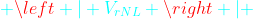or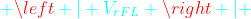means the values or magnitudes of  voltages without sign . So the subtraction is the simple subtraction and not the phasor subtraction. The voltage regulation is desired to be small or zero.  Integrated Grids has set standards  for voltage variation say  ±5% or ±7% depending on voltage levels.

The relationship  Vs = Vr  + Z Ir   between the sending end and receiving end voltages can also be illustrated by a phasor diagram shown in Fig-B. In Fig-B(i) we have considered an inductive load. So the receiving end current  Ir   lags the receiving end voltage Vr by a phase angle φ(determined by load). In Fig-B(ii) is considered a capacitive load. Here current  Ir  leads the voltage Vby an angle φ.

In both the diagrams Vr  and  Z Ir   are added as per the rule of phasor addition. Z is the line impedance as discussed above.

Z I = (R+j XL ) I = R Ir   +j XL I

The main difference between the above two is that in case of lagging load the receiving end voltage is less than the sending end voltage. But in case of leading load the receiving end voltage can even become more than the sending end voltage depending on the lead phase angle φ . Which is due to the fact that the load is capacitive. In our case Fig-B (ii), for the chosen phase angle the receiving end voltage is more than the sending end voltage. Actually the industrial loads are mostly inductive. The inductive loads consume reactive power. Capacitors which generate reactive power are connected at the load end or at substations to supply reactive power. At sub-transmission and distribution levels where voltage drop is a problem, capacitors can improve the voltage profile and power factor at the receiving end. The capacitive load helps in improving the receiving end voltage regulation and power factor. For only voltage regulation purpose usually tap changer of transformer is utilized. By tap changing the transformer turns ratio is changed, so adjusting the secondary voltage.

Another common definition which sometimes may be useful for evaluation of transmission line performance is the transmission efficiency of the line. The transmission efficiency is defined as the ratio of power delivered at receiving end to the power supplied at sending end.

If η is the transmission efficiency then,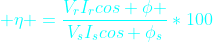cos φ  is the power factor at the receiving end, or power factor of load but  cos φ is the power factor at the sending end (due to both load and transmission line).
Using the above formula it is easy to find the transmission efficiency of short and medium lines. For short line the angle φs is clearly visible from the phasor diagram (angle between  Vs and Is (or Ir)). But in case of medium line little more effort is required.

Medium Lines

The medium length lines are categorized as transmission lines of more than 80 km and less than 250 km length. The medium length lines can be modeled two ways. These are nominal π and nomial T (Fig-C), so named due to the arrangement of elements. Due to the length of the  line admittance Y is no more neglected. Of course in both the π and nomial T representations lumped elements are used.  In the nominal π representation the total admittance due to line capacitance is equally divided into two halves and each half connected at both sending and receiving ends. In the nominal T representation the admittance is connected at the center of line and line impedance is divided into two equal parts and each part is connected on both sides in series (Fig-C).

It can be shown that for the π representation the sending end voltage and current can be written in terms of receiving end voltage and current as shown below.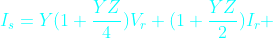From above two expressions ABCD parameters can be easily identified. Also you should verify the condition AD-BC=1.

For T representation also applying KCL and KVL we can obtain the similar expression for Vs and Is.

At no load putting I= 0   we obtain Vr NL  = V/ A, .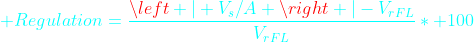Analysis of short and medium lines helps us gain important concepts of transmission lines. It is useful for long line analysis.

### Influence of Earth on Capacitance Calculation

In last article we discussed the technique for calculation of inductance and capacitance of double circuit transmission line, which can be easily applied for multi circuit lines. Of course before proceeding further I strongly recommend to go through those articles. I also advise to have a look at the two articles on calculation of line inductance and capacitance in general. Moreover as this article uses basic formulas of logarithm so we have tabulated few useful formulas at the bottom.

In the above three articles we developed some basic concepts. In the previous articles we neglected the influence of earth on capacitance calculation of transmission lines. But this is not true for Extra High voltage (EHV) and Ultra High Voltage (UHV) lines. For example for transmission lines above 200 kV voltage level the clearance distance between phases is quite comparable with the clearance between phase and ground. And electric field is considerably influenced by the presence of earth. This situation requires the consideration of influence of earth on calculation of line capacitance.

Here I will derive the formulas for calculation of transmission line capacitance.

Let us consider a charged line of circular cross section conductor as shown in Fig A. When this object is far removed from earth its electric field will be as shown in Fig-A(i), radial lines of force. In Fig-A(ii) is shown how the electric field is disturbed in the presence of Earth.  This field pattern above ground can be obtained by replacing the ground and placing an imaginary  oppositely charged similar conductor  same distance below the ground line. Here it is imagined that the ground level as a mirror. This is called image charge (see Fig-A (iii)). Now the problem is reduced to a pair of opposite charges.

Let us consider Fig-B where there are two very long line conductors of charge +q and -q coulmb per meter length of the conductor. From the basics it can be shown that the potential at a point P is vp
Then,

The above equation can also be written as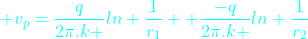The above equation can be imagined as the potential ( vp) at the point ' p ' is composed of two components one due to positive charge +q and other due to negative charge -q. These two are respectively first and second terms in the above equation.

Generalizing it we can say that the total potential at a point p due to several charges is equal to the sum of the potential due to all the individual charges.

Here next we will consider our favorite and practical case of the three phase line. Let us assume that the three phase conductors are placed in a general arbitrary form as shown in Fig -C. Now imagining the ground level as mirror we get the image charges which are -ve of actual charges on conductors as shown. Now the ground line can be removed. In the figure all the distances between the conductors and/or image are illustrated.

As illustrated in above case we will obtain the voltage difference between the conductors a and its image a' . For this we have to find the potential at the surface of a  and a'. Let the potential at a is va and the potential at a' is va'.

As already said the potential at the surface of conductor a is the simple algebraic sum of the potential due to charges on all the conductors including this particular conductor a. Similarly we can find the potential at the surface of a'.

This way we get,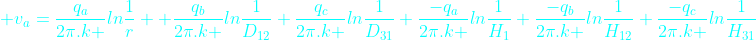Now Vaa' = va-va'

So from the above two equations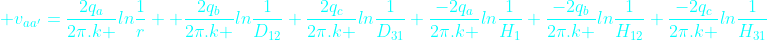Or in compact form we can write as

As already said the phase conductors are transposed. So for ⅓ rd of distance the conductor of  phase-a will take the position of  phase-b and conductor of phase-b will take that of  c .  So for all the three positions we will calculate the voltage for ' a ' phase and take the average. As already said in last articles  by transposition, the capacitance between any of the three conductors and neutral are made equal. If C denotes capacitance then after transposition we get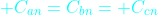Of course here we will concentrate on calculation of capacitance (Can) between phase-a  and neutral.

Due to transposition when the phase a conductors will take position of b as shown above and of course b will take position of c and c will take that of a. (You can think cyclically).

Similarly when  phase-a   conductor occupies the other position .

Let us call the average voltage between conductor a and image conductor a' be va-a'
Then,

Combining above three we get the value of va-a'
But we require the voltage from neutral (n) to phase-a conductor (see Fig-D), that is van

From Fig-D it is clear that,

Hence we get

Simplifying we get

or,

but the charges of three phases sum up to zero,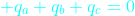So,

by rearranging the terms (see the properties of logarithm below at the end) we finally get

We know that the capacitance between phase a and neutral Can is,

So finally we get

From the above derived formula of capacitance Can  we observe that due to the consideration of influence of earth the denominator second term comes into picture and the capacitance Can  is more than what we would have got if earth influence was ignored.

Logarithm Formulas Table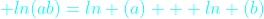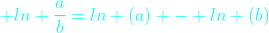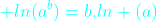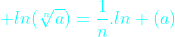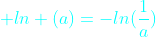Here ln means natural logarithm. Natural logarithm is the logarithm when the base is e. The value of e is approximately 2.718.

### Double Circuit Inductance and Capacitance

Introduction

In last two articles we developed some useful concepts on transmission line inductance and capacitance. There we discussed the formulas used for calculation of inductance and capacitance of transmission lines for different arrangement of conductors. I advise you to go through those two topics in archive (above links) before proceeding further. In those two articles our discussion was restricted to single phase or one three phase line (single circuit line).  Here we will calculate the inductance and capacitance of double circuit line which you can extend to multi-circuits.
Double circuit GMD and GMR

In high voltage transmission system you will often find transmission towers carrying two or more circuits. In fact numerous multi-circuit lines are already in use. The conductors of circuits are arranged in different configuration depending on the  tower types used. We will calculate inductance for a double circuit line using the formula.

Although the two circuits are in parallel, the inductance of double circuit can not be found by imagining two inductances(for two circuits) in parallel. Actually this is true only  when the magnetic field of one circuit does not link with other circuit conductors.  Hence that is the case when each circuit runs on separate tower and the separation between them is such so that there is negligible or no magnetic field interaction between the circuits.

Due to the closeness of the circuits being on the same tower, the  magnetic and electric field interaction of one circuit on the other requires modified calculation of GMD and GMR.

See the Figure-A below for a double circuit arrangement on a self supporting lattice tower. Here we have chosen the conductors arranged vertically which will simplify our calculation and we will derive the inductance for this arrangement where the phase conductors are also assumed as transposed. As we discuss the topic you will realize that the method can be applied to other arrangements or multi-circuits also.

In the figure one circuit phase conductors are a-b-c and other circuit phase conductors are a'-b'-c'. As shown here  the phase conductors are single rounded conductors.

In the last two articles we have already discussed bundled conductors for inductance and capacitance calculation. Here the double circuit is treated very similar to single circuit or a three phase line with bundled conductors. The conductors a-a' are imagined as bundle conductor for phase A, similarly b-b' and c-c' are imagined as bundle conductors for phases B and C. Of course here the bundle sub-conductors are far away and not bunched unlike the case of twin, triple or quad conductors bundles.

Now we are ready to apply the general formula for calculating Geometric Mean Distance(GMD). For calculating GMD all the distances between the phase conductors are identified. In Fig-A(ii) all the distances between the phases are shown. For example a-b, a-c, a-c', a-b', c-b', c-a' etc.. There are 12 possible distances between conductors of phases as shown. It should be noted that for calculation of GMD the distances a-a', b-b' and c-c' are not taken.

GMD = (Dab . Dab' . Dbc . Dbc' . Dca . Dca' . Da'b . Da'b' . Db'c . Db'c' . Dc'a . Dc'a')1/12

Clearly there are 12 distances and so 12th root of the product of twelve distances are taken.

By rearranging the terms you can also write GMD as below.

GMD = (DAB . DBC . DCA.)1/3

Where DAB = (Dab . Dab' . Da'b . Da'b')1/4   similarly DBC and DCA.

In our example

GMD = (6 . 10 . 6 . 10 . 12 . 8 . 10 . 6 . 10 . 6 . 8 . 12)1/12  meter
= 8.37  meter.

Now let us calculate the Geometric Mean Radius (GMR)

For GMR calculation the method is just similar to bundle conductors. Of course for inductance calculation we require r' and for capacitance r. As already said in previous articles the equivalent radius r' =0.7788r .
Here let us first calculate the GMR of each phase separately for inductance.

GMR= √(Daa' . r')

GMR= √(Dbb' . r')

GMR= √(Dcc' . r')

Daa'  is the distance between the conductors a and a'. Similarly for Dbb'   and  Dcc'

As the phases are transposed so,
GMRL = (GMRa  . GMR. GMRc)1/3

Note: Daa'  Dbb'   and  Dcc'   were not used in GMD calculation but used in GMRL  calculation.
Subscript L is used for GMR of inductance calculation and subscript C for capacitance.

Putting the values of GMD and GMRL   in the equation

L = 2 * 10 -7 ln ( GMD/GMR)

we obtain inductance per meter per phase

In above calculation for GMRL the conductor is circular of radius r. But in actual practice ACSR conductors are mostly used, so for inductance calculation r' is replaced by Ds as supplied by the manufacturer of ACSR conductor.
For capacitance calculation the radius of conductor is used in GMRC formula whether it is one circular conductor or ACSR conductor, for calculation of capacitance,

GMR= √(Daa' . r)

GMR= √(Dbb' . r)

GMR= √(Dcc' . r)

GMRC = (GMRa  . GMR. GMRc)1/3

putting the value of  GMR in the equation,
Cn = 2pk / ln (GMD/ GMRc)

We obtain the value of capacitance ( Cn ) per meter between phase and neutral .
k is the permittivity of air and GMD is already calculated for inductance.

Now you can put the numerical values to get a feel of the Cn so obtained.